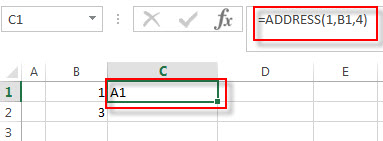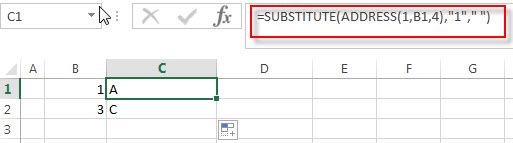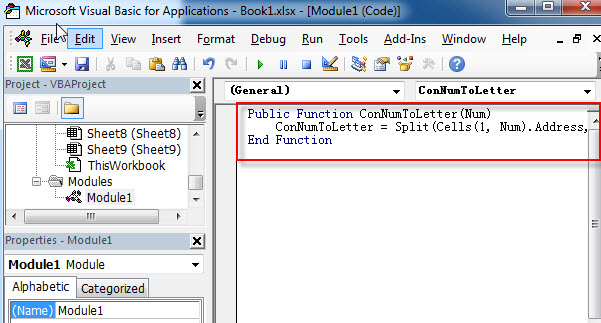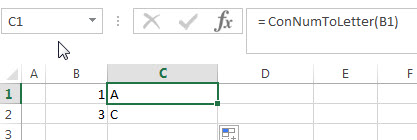# How to convert column number to letter

This post explains that how to convert a column number to a column letter using formula in excel. How to convert column number to a column letter using user defined function in VBA.

## Convert column number to letter using excel formula

if you want to convert column number to letter, you can use the ADDRESS function to get the absolute reference of one excel cell that contains that column number, such as, you can get the address of Cell B1, then you can use the SUBSTITUTE function to replace row number with empty string, so that you can get the column letter. So you can write down the following formula using the SUBSTITUTE function and the ADDRESS function.

`=SUBSTITUTE(ADDRESS(1,B1,4),"1"," ")`

Let’s see how this formula works:The ADDRESS function returns an absolute reference for a cell at a given row and column number. And this formula returns A1. The returned address goes into the SUBSTITUTE function as its Text argument.This formula will replace the old_text “1” with empty string for a string of cell reference returned by the ADDRESS. So it returns a letter.

## Convert column number to letter with VBA user defind function

You can also create a new user defined function to convert column number to  a column letter in Excel VBA:

1# click on “Visual Basic” command under DEVELOPER Tab.2# then the “Visual Basic Editor” window will appear.

3# click “Insert” ->”Module” to create a new module named4# paste the below VBA code into the code window. Then clicking “Save” button.

```Public Function ConNumToLetter(Collet)
End Function```5# back to the current worksheet, then enter the below formula in Cell C1:

`= ConNumToLetter(B1)`### Related Formulas

• Extract text after first comma or space
If you want to get substring after the first comma character from a text string in Cell B1, then you can create a formula based on the MID function and FIND function or SEARCH function ….
• Convert column letter to number
If you want to convert a column letter to number, you can use a combination of the COLUMN function and the INDIRECT function to create an excel formula…..